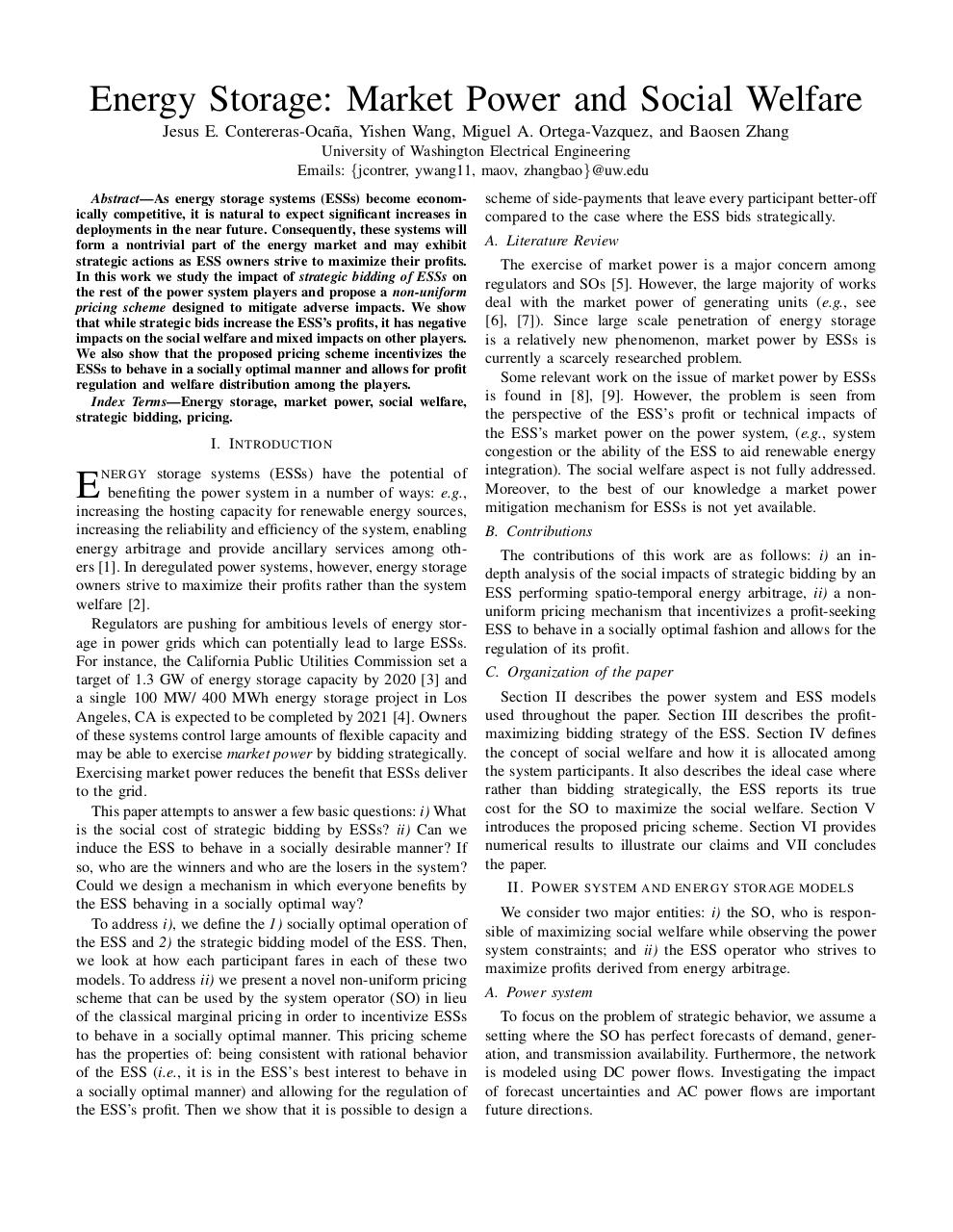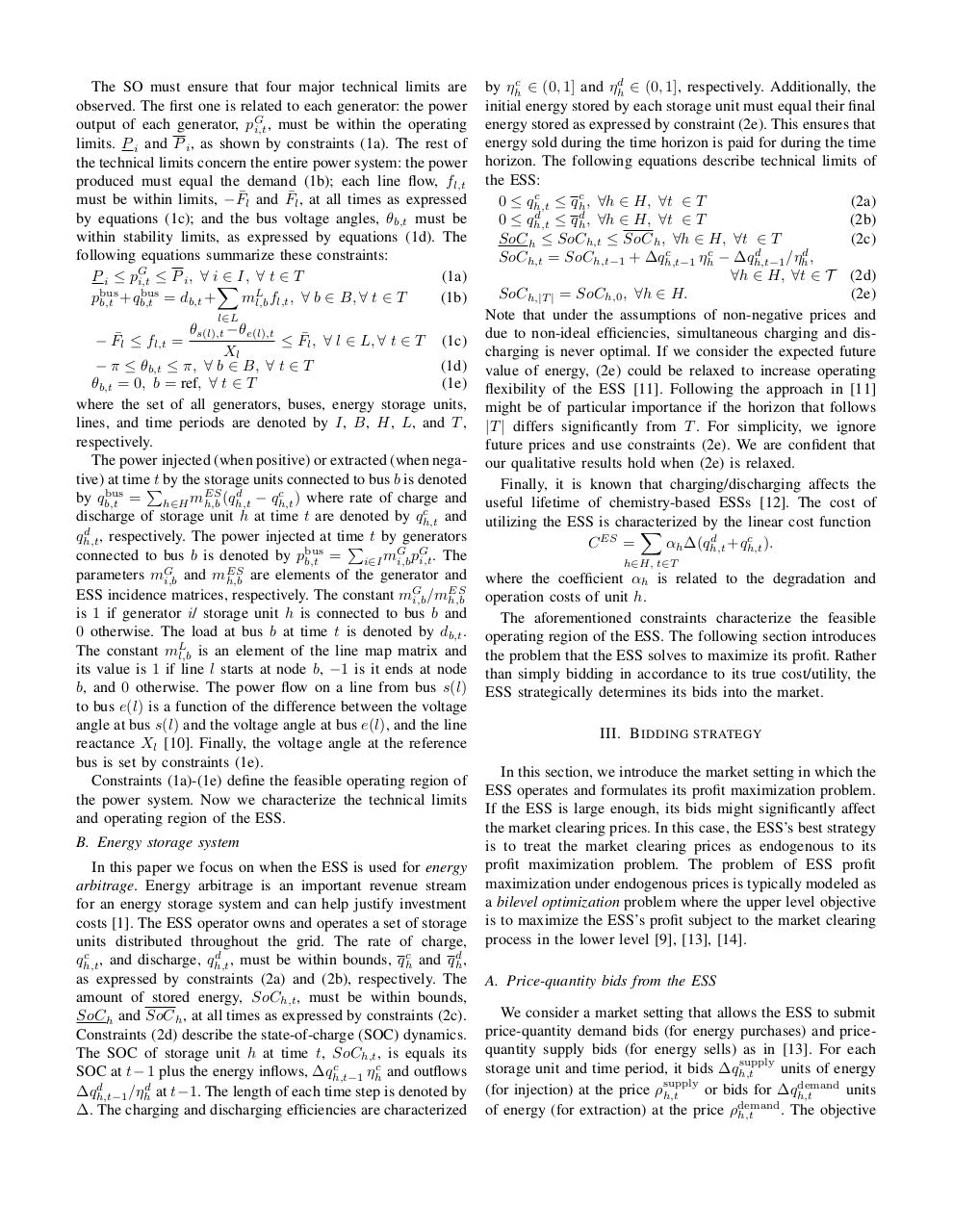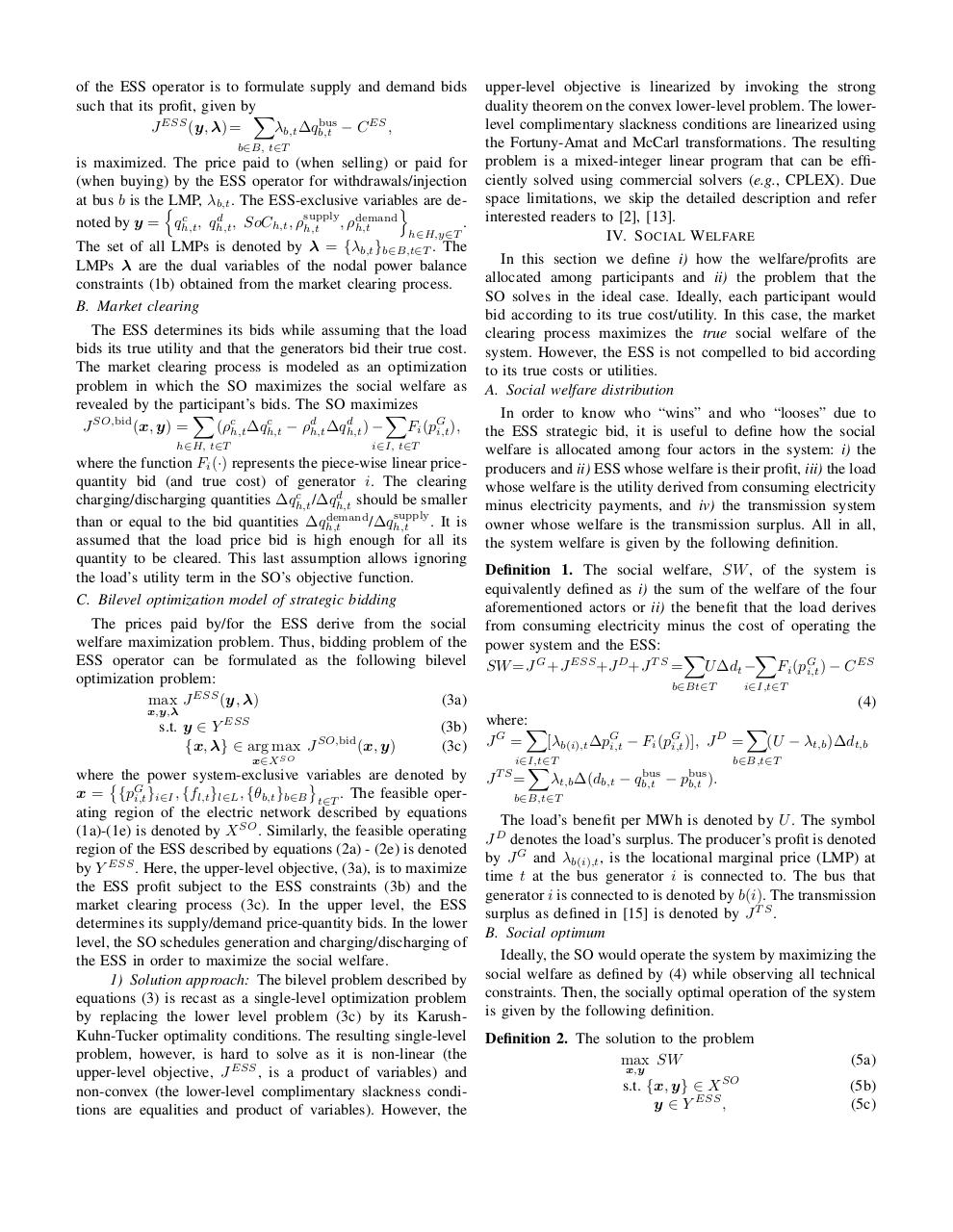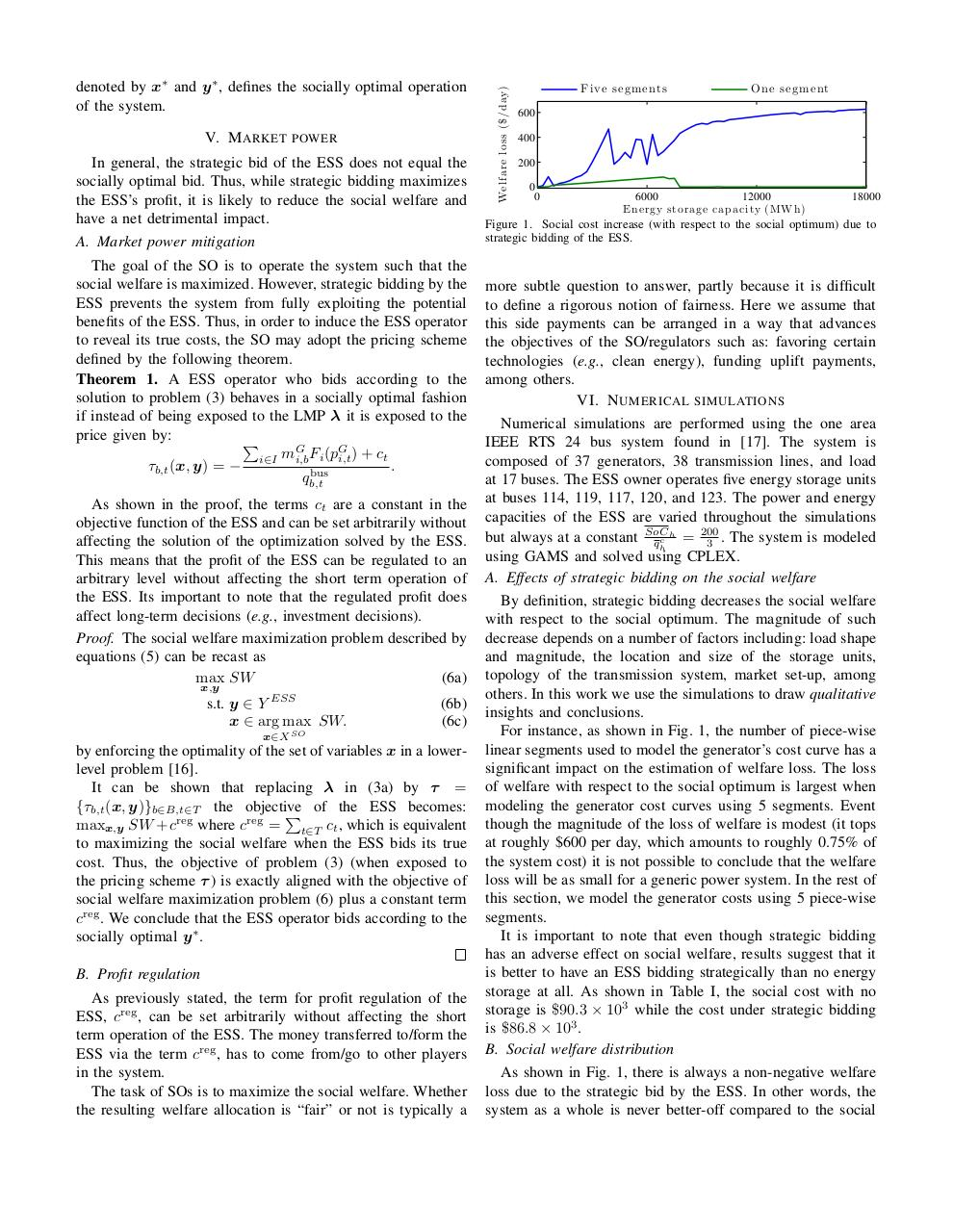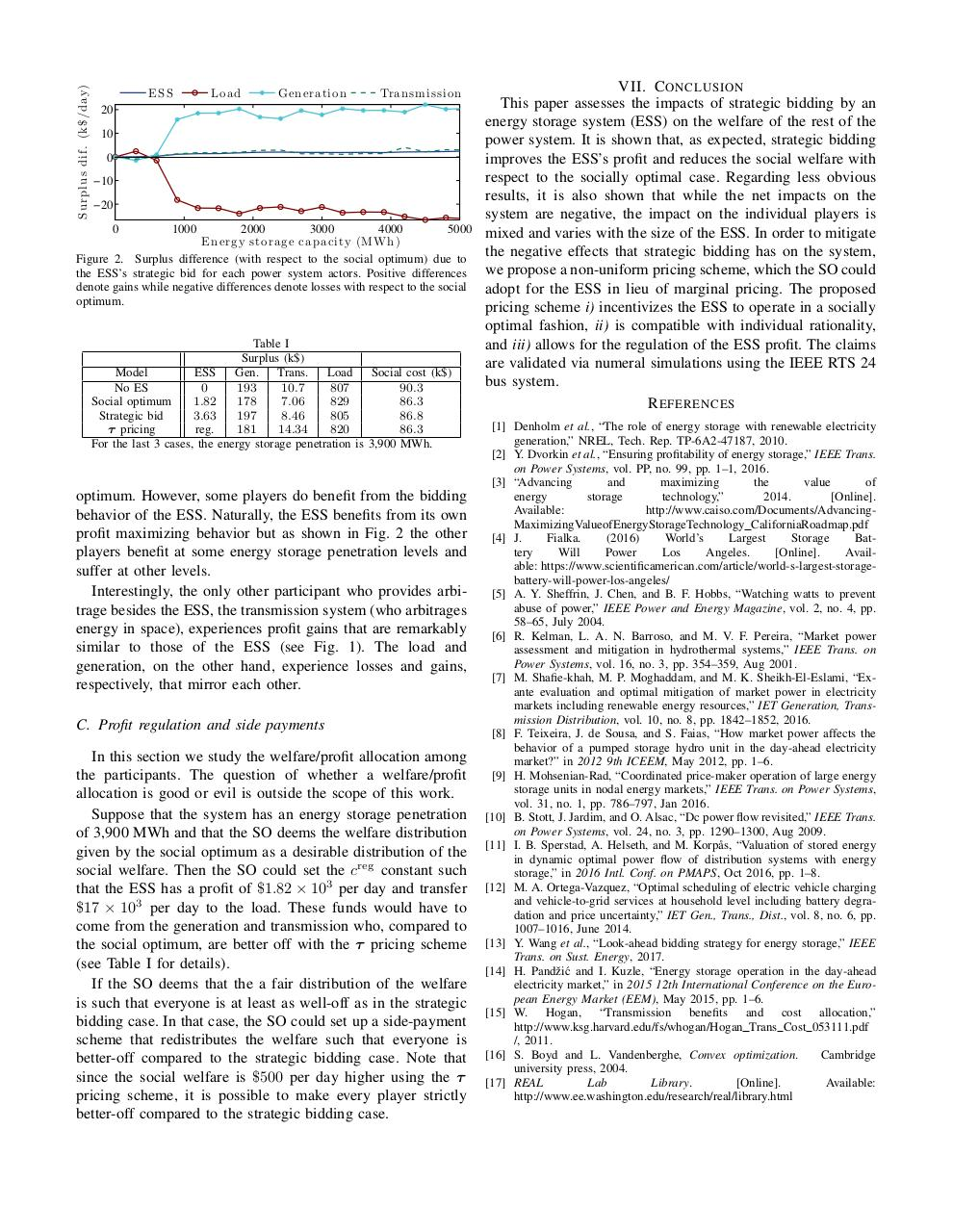# Energy storage market power and social welfare .pdf

### File information

Original filename: Energy storage market power and social welfare.pdf

This PDF 1.5 document has been generated by TeX / pdfTeX-1.40.16, and has been sent on pdf-archive.com on 13/11/2017 at 03:58, from IP address 97.113.x.x. The current document download page has been viewed 205 times.
File size: 228 KB (5 pages).
Privacy: public file

Energy storage market power and social welfare.pdf (PDF, 228 KB)

### Document preview

Energy Storage: Market Power and Social Welfare
Jesus E. Contereras-Oca˜na, Yishen Wang, Miguel A. Ortega-Vazquez, and Baosen Zhang
University of Washington Electrical Engineering
Emails: {jcontrer, ywang11, maov, zhangbao}@uw.edu
Abstract—As energy storage systems (ESSs) become economically competitive, it is natural to expect significant increases in
deployments in the near future. Consequently, these systems will
form a nontrivial part of the energy market and may exhibit
strategic actions as ESS owners strive to maximize their profits.
In this work we study the impact of strategic bidding of ESSs on
the rest of the power system players and propose a non-uniform
pricing scheme designed to mitigate adverse impacts. We show
that while strategic bids increase the ESS’s profits, it has negative
impacts on the social welfare and mixed impacts on other players.
We also show that the proposed pricing scheme incentivizes the
ESSs to behave in a socially optimal manner and allows for profit
regulation and welfare distribution among the players.
Index Terms—Energy storage, market power, social welfare,
strategic bidding, pricing.

I. I NTRODUCTION
storage systems (ESSs) have the potential of
benefiting the power system in a number of ways: e.g.,
increasing the hosting capacity for renewable energy sources,
increasing the reliability and efficiency of the system, enabling
energy arbitrage and provide ancillary services among others . In deregulated power systems, however, energy storage
owners strive to maximize their profits rather than the system
welfare .
Regulators are pushing for ambitious levels of energy storage in power grids which can potentially lead to large ESSs.
For instance, the California Public Utilities Commission set a
target of 1.3 GW of energy storage capacity by 2020  and
a single 100 MW/ 400 MWh energy storage project in Los
Angeles, CA is expected to be completed by 2021 . Owners
of these systems control large amounts of flexible capacity and
may be able to exercise market power by bidding strategically.
Exercising market power reduces the benefit that ESSs deliver
to the grid.
This paper attempts to answer a few basic questions: i) What
is the social cost of strategic bidding by ESSs? ii) Can we
induce the ESS to behave in a socially desirable manner? If
so, who are the winners and who are the losers in the system?
Could we design a mechanism in which everyone benefits by
the ESS behaving in a socially optimal way?
To address i), we define the 1) socially optimal operation of
the ESS and 2) the strategic bidding model of the ESS. Then,
we look at how each participant fares in each of these two
models. To address ii) we present a novel non-uniform pricing
scheme that can be used by the system operator (SO) in lieu
of the classical marginal pricing in order to incentivize ESSs
to behave in a socially optimal manner. This pricing scheme
has the properties of: being consistent with rational behavior
of the ESS (i.e., it is in the ESS’s best interest to behave in
a socially optimal manner) and allowing for the regulation of
the ESS’s profit. Then we show that it is possible to design a

E

NERGY

scheme of side-payments that leave every participant better-off
compared to the case where the ESS bids strategically.
A. Literature Review
The exercise of market power is a major concern among
regulators and SOs . However, the large majority of works
deal with the market power of generating units (e.g., see
, ). Since large scale penetration of energy storage
is a relatively new phenomenon, market power by ESSs is
currently a scarcely researched problem.
Some relevant work on the issue of market power by ESSs
is found in , . However, the problem is seen from
the perspective of the ESS’s profit or technical impacts of
the ESS’s market power on the power system, (e.g., system
congestion or the ability of the ESS to aid renewable energy
integration). The social welfare aspect is not fully addressed.
Moreover, to the best of our knowledge a market power
mitigation mechanism for ESSs is not yet available.
B. Contributions
The contributions of this work are as follows: i) an indepth analysis of the social impacts of strategic bidding by an
ESS performing spatio-temporal energy arbitrage, ii) a nonuniform pricing mechanism that incentivizes a profit-seeking
ESS to behave in a socially optimal fashion and allows for the
regulation of its profit.
C. Organization of the paper
Section II describes the power system and ESS models
used throughout the paper. Section III describes the profitmaximizing bidding strategy of the ESS. Section IV defines
the concept of social welfare and how it is allocated among
the system participants. It also describes the ideal case where
rather than bidding strategically, the ESS reports its true
cost for the SO to maximize the social welfare. Section V
introduces the proposed pricing scheme. Section VI provides
numerical results to illustrate our claims and VII concludes
the paper.
II. P OWER SYSTEM AND ENERGY STORAGE MODELS
We consider two major entities: i) the SO, who is responsible of maximizing social welfare while observing the power
system constraints; and ii) the ESS operator who strives to
maximize profits derived from energy arbitrage.
A. Power system
To focus on the problem of strategic behavior, we assume a
setting where the SO has perfect forecasts of demand, generation, and transmission availability. Furthermore, the network
is modeled using DC power flows. Investigating the impact
of forecast uncertainties and AC power flows are important
future directions.

The SO must ensure that four major technical limits are
observed. The first one is related to each generator: the power
output of each generator, pG
i,t , must be within the operating
limits. P i and P i , as shown by constraints (1a). The rest of
the technical limits concern the entire power system: the power
produced must equal the demand (1b); each line flow, fl,t
must be within limits, −F¯l and F¯l , at all times as expressed
by equations (1c); and the bus voltage angles, θb,t must be
within stability limits, as expressed by equations (1d). The
following equations summarize these constraints:
∈ I, ∀ t ∈ T
(1a)
P i ≤ pG
i,t ≤ P i , ∀ iX
bus
L
pbus
+q
=
d
+
m
f
,

b

B,

t

T
(1b)
b,t
b,t
b,t
l,b l,t
l∈L

θs(l),t −θe(l),t
− F¯l ≤ fl,t =
≤ F¯l , ∀ l ∈ L, ∀ t ∈ T (1c)
Xl
− π ≤ θb,t ≤ π, ∀ b ∈ B, ∀ t ∈ T
(1d)
θb,t = 0, b = ref, ∀ t ∈ T
(1e)
where the set of all generators, buses, energy storage units,
lines, and time periods are denoted by I, B, H, L, and T ,
respectively.
The power injected (when positive) or extracted (when negative) at timeP
t by the storage units connected to bus b is denoted
bus
d
c
by qb,t
= h∈H mES
h,b (qh,t − qh,t ) where rate of charge and
c
discharge of storage unit h at time t are denoted by qh,t
and
d
qh,t , respectively. The power injected at time t by generators
P
G G
connected to bus b is denoted by pbus
b,t =
i∈I mi,b pi,t . The
G
ES
parameters mi,b and mh,b are elements of the generator and
ES
ESS incidence matrices, respectively. The constant mG
i,b /mh,b
is 1 if generator i/ storage unit h is connected to bus b and
0 otherwise. The load at bus b at time t is denoted by db,t .
The constant mL
l,b is an element of the line map matrix and
its value is 1 if line l starts at node b, −1 is it ends at node
b, and 0 otherwise. The power flow on a line from bus s(l)
to bus e(l) is a function of the difference between the voltage
angle at bus s(l) and the voltage angle at bus e(l), and the line
reactance Xl . Finally, the voltage angle at the reference
bus is set by constraints (1e).
Constraints (1a)-(1e) define the feasible operating region of
the power system. Now we characterize the technical limits
and operating region of the ESS.
B. Energy storage system
In this paper we focus on when the ESS is used for energy
arbitrage. Energy arbitrage is an important revenue stream
for an energy storage system and can help justify investment
costs . The ESS operator owns and operates a set of storage
units distributed throughout the grid. The rate of charge,
c
d
qh,t
, and discharge, qh,t
, must be within bounds, q ch and q dh ,
as expressed by constraints (2a) and (2b), respectively. The
amount of stored energy, SoCh,t , must be within bounds,
SoC h and SoC h , at all times as expressed by constraints (2c).
Constraints (2d) describe the state-of-charge (SOC) dynamics.
The SOC of storage unit h at time t, SoCh,t , is equals its
c
SOC at t−1 plus the energy inflows, ∆qh,t−1
ηhc and outflows
d
d
∆qh,t−1 /ηh at t−1. The length of each time step is denoted by
∆. The charging and discharging efficiencies are characterized

by ηhc ∈ (0, 1] and ηhd ∈ (0, 1], respectively. Additionally, the
initial energy stored by each storage unit must equal their final
energy stored as expressed by constraint (2e). This ensures that
energy sold during the time horizon is paid for during the time
horizon. The following equations describe technical limits of
the ESS:
c
(2a)
0 ≤ qh,t
≤ q ch , ∀h ∈ H, ∀t ∈ T
d
(2b)
0 ≤ qh,t ≤ q dh , ∀h ∈ H, ∀t ∈ T
SoC h ≤ SoCh,t ≤ SoC h , ∀h ∈ H, ∀t ∈ T
(2c)
c
d
SoCh,t = SoCh,t−1 + ∆qh,t−1
ηhc − ∆qh,t−1
/ηhd ,
∀h ∈ H, ∀t ∈ T (2d)
SoCh,|T | = SoCh,0 , ∀h ∈ H.
(2e)
Note that under the assumptions of non-negative prices and
due to non-ideal efficiencies, simultaneous charging and discharging is never optimal. If we consider the expected future
value of energy, (2e) could be relaxed to increase operating
flexibility of the ESS . Following the approach in 
might be of particular importance if the horizon that follows
|T | differs significantly from T . For simplicity, we ignore
future prices and use constraints (2e). We are confident that
our qualitative results hold when (2e) is relaxed.
Finally, it is known that charging/discharging affects the
useful lifetime of chemistry-based ESSs . The cost of
utilizing the ESS is characterized
by the linear cost function
X
ES
d
c
C
=
αh ∆(qh,t
+qh,t
).
h∈H, t∈T

where the coefficient αh is related to the degradation and
operation costs of unit h.
The aforementioned constraints characterize the feasible
operating region of the ESS. The following section introduces
the problem that the ESS solves to maximize its profit. Rather
than simply bidding in accordance to its true cost/utility, the
ESS strategically determines its bids into the market.
III. B IDDING STRATEGY
In this section, we introduce the market setting in which the
ESS operates and formulates its profit maximization problem.
If the ESS is large enough, its bids might significantly affect
the market clearing prices. In this case, the ESS’s best strategy
is to treat the market clearing prices as endogenous to its
profit maximization problem. The problem of ESS profit
maximization under endogenous prices is typically modeled as
a bilevel optimization problem where the upper level objective
is to maximize the ESS’s profit subject to the market clearing
process in the lower level , , .
A. Price-quantity bids from the ESS
We consider a market setting that allows the ESS to submit
price-quantity demand bids (for energy purchases) and pricequantity supply bids (for energy sells) as in . For each
supply
storage unit and time period, it bids ∆qh,t
units of energy
supply
demand
(for injection) at the price ρh,t
or bids for ∆qh,t
units
demand
of energy (for extraction) at the price ρh,t
. The objective

of the ESS operator is to formulate supply and demand bids
such that its profit, given byX
bus
J ESS (y, λ) =
λb,t ∆qb,t
− C ES ,
b∈B, t∈T

is maximized. The price paid to (when selling) or paid for
(when buying) by the ESS operator for withdrawals/injection
at bus b is thenLMP, λb,t . The ESS-exclusive variables
o are desupply demand
c
d
noted by y = qh,t , qh,t , SoCh,t , ρh,t , ρh,t
.
h∈H,y∈T

The set of all LMPs is denoted by λ = {λb,t }b∈B,t∈T . The
LMPs λ are the dual variables of the nodal power balance
constraints (1b) obtained from the market clearing process.
B. Market clearing
The ESS determines its bids while assuming that the load
bids its true utility and that the generators bid their true cost.
The market clearing process is modeled as an optimization
problem in which the SO maximizes the social welfare as
revealed by the participant’s
bids. The SO maximizes
X
X
SO,bid
c
c
d
J
(x, y) =
(ρh,t ∆qh,t
− ρdh,t ∆qh,t
)−
Fi (pG
i,t ),
i∈I, t∈T

h∈H, t∈T

where the function Fi (·) represents the piece-wise linear pricequantity bid (and true cost) of generator i. The clearing
c
d
charging/discharging quantities ∆qh,t
/∆qh,t
should be smaller
supply
demand
than or equal to the bid quantities ∆qh,t
/∆qh,t
. It is
assumed that the load price bid is high enough for all its
quantity to be cleared. This last assumption allows ignoring
the load’s utility term in the SO’s objective function.
C. Bilevel optimization model of strategic bidding
The prices paid by/for the ESS derive from the social
welfare maximization problem. Thus, bidding problem of the
ESS operator can be formulated as the following bilevel
optimization problem:
max J ESS (y, λ)
(3a)
x,y,λ

ESS

s.t. y ∈ Y
{x, λ} ∈ arg max J SO,bid (x, y)

(3b)
(3c)

x∈X SO

where the power system-exclusive variables
are denoted by

x = {pG
i,t }i∈I , {fl,t }l∈L , {θb,t }b∈B t∈T . The feasible operating region of the electric network described by equations
(1a)-(1e) is denoted by X SO . Similarly, the feasible operating
region of the ESS described by equations (2a) - (2e) is denoted
by Y ESS . Here, the upper-level objective, (3a), is to maximize
the ESS profit subject to the ESS constraints (3b) and the
market clearing process (3c). In the upper level, the ESS
determines its supply/demand price-quantity bids. In the lower
level, the SO schedules generation and charging/discharging of
the ESS in order to maximize the social welfare.
1) Solution approach: The bilevel problem described by
equations (3) is recast as a single-level optimization problem
by replacing the lower level problem (3c) by its KarushKuhn-Tucker optimality conditions. The resulting single-level
problem, however, is hard to solve as it is non-linear (the
upper-level objective, J ESS , is a product of variables) and
non-convex (the lower-level complimentary slackness conditions are equalities and product of variables). However, the

upper-level objective is linearized by invoking the strong
duality theorem on the convex lower-level problem. The lowerlevel complimentary slackness conditions are linearized using
the Fortuny-Amat and McCarl transformations. The resulting
problem is a mixed-integer linear program that can be efficiently solved using commercial solvers (e.g., CPLEX). Due
space limitations, we skip the detailed description and refer
IV. S OCIAL W ELFARE
In this section we define i) how the welfare/profits are
allocated among participants and ii) the problem that the
SO solves in the ideal case. Ideally, each participant would
bid according to its true cost/utility. In this case, the market
clearing process maximizes the true social welfare of the
system. However, the ESS is not compelled to bid according
to its true costs or utilities.
A. Social welfare distribution
In order to know who “wins” and who “looses” due to
the ESS strategic bid, it is useful to define how the social
welfare is allocated among four actors in the system: i) the
producers and ii) ESS whose welfare is their profit, iii) the load
whose welfare is the utility derived from consuming electricity
minus electricity payments, and iv) the transmission system
owner whose welfare is the transmission surplus. All in all,
the system welfare is given by the following definition.
Definition 1. The social welfare, SW , of the system is
equivalently defined as i) the sum of the welfare of the four
aforementioned actors or ii) the benefit that the load derives
from consuming electricity minus the cost of operating the
power system and the ESS: X
X
ES
SW= J G +J ESS+J D+J T S = U ∆dt − Fi (pG
i,t ) − C
i∈I,t∈T

b∈Bt∈T

(4)
where:X
X
G
D
JG =
[λb(i),t ∆pG
= (U − λt,b )∆dt,b
i,t − Fi (pi,t )], J
i∈I,t∈T
b∈B,t∈T
X
bus
J T S=
λt,b ∆(db,t − qb,t
− pbus
).
b,t
b∈B,t∈T

The load’s benefit per MWh is denoted by U . The symbol
J D denotes the load’s surplus. The producer’s profit is denoted
by J G and λb(i),t , is the locational marginal price (LMP) at
time t at the bus generator i is connected to. The bus that
generator i is connected to is denoted by b(i). The transmission
surplus as defined in  is denoted by J T S .
B. Social optimum
Ideally, the SO would operate the system by maximizing the
social welfare as defined by (4) while observing all technical
constraints. Then, the socially optimal operation of the system
is given by the following definition.
Definition 2. The solution to the problem
max SW
x,y

SO

s.t. {x, y} ∈ X
y ∈ Y ESS ,

(5a)
(5b)
(5c)

V. M ARKET POWER
In general, the strategic bid of the ESS does not equal the
socially optimal bid. Thus, while strategic bidding maximizes
the ESS’s profit, it is likely to reduce the social welfare and
have a net detrimental impact.
A. Market power mitigation
The goal of the SO is to operate the system such that the
social welfare is maximized. However, strategic bidding by the
ESS prevents the system from fully exploiting the potential
benefits of the ESS. Thus, in order to induce the ESS operator
to reveal its true costs, the SO may adopt the pricing scheme
defined by the following theorem.
Theorem 1. A ESS operator who bids according to the
solution to problem (3) behaves in a socially optimal fashion
if instead of being exposed to the LMP λ it is exposed to the
price given by:
P
G
G
i∈I mi,b Fi (pi,t ) + ct
.
τb,t (x, y) = −
bus
qb,t
As shown in the proof, the terms ct are a constant in the
objective function of the ESS and can be set arbitrarily without
affecting the solution of the optimization solved by the ESS.
This means that the profit of the ESS can be regulated to an
arbitrary level without affecting the short term operation of
the ESS. Its important to note that the regulated profit does
affect long-term decisions (e.g., investment decisions).
Proof. The social welfare maximization problem described by
equations (5) can be recast as
max SW
(6a)
x,y

s.t. y ∈ Y ESS
x ∈ arg max SW.

(6b)
(6c)

x∈X SO

by enforcing the optimality of the set of variables x in a lowerlevel problem .
It can be shown that replacing λ in (3a) by τ =
{τb,t (x, y)}b∈B,t∈T the objective
P of the ESS becomes:
maxx,y SW +creg where creg = t∈T ct , which is equivalent
to maximizing the social welfare when the ESS bids its true
cost. Thus, the objective of problem (3) (when exposed to
the pricing scheme τ ) is exactly aligned with the objective of
social welfare maximization problem (6) plus a constant term
creg . We conclude that the ESS operator bids according to the
socially optimal y ∗ .
B. Profit regulation
As previously stated, the term for profit regulation of the
ESS, creg , can be set arbitrarily without affecting the short
term operation of the ESS. The money transferred to/form the
ESS via the term creg , has to come from/go to other players
in the system.
The task of SOs is to maximize the social welfare. Whether
the resulting welfare allocation is “fair” or not is typically a

Wel fare l oss (\$/d ay)

denoted by x∗ and y ∗ , defines the socially optimal operation
of the system.

Five s e gme nt s

One s e gme nt

600
400
200
0
0

6000
12000
E n ergy storage cap aci ty (MW h )

18000

Figure 1. Social cost increase (with respect to the social optimum) due to
strategic bidding of the ESS.

more subtle question to answer, partly because it is difficult
to define a rigorous notion of fairness. Here we assume that
this side payments can be arranged in a way that advances
the objectives of the SO/regulators such as: favoring certain
technologies (e.g., clean energy), funding uplift payments,
among others.
VI. N UMERICAL SIMULATIONS
Numerical simulations are performed using the one area
IEEE RTS 24 bus system found in . The system is
composed of 37 generators, 38 transmission lines, and load
at 17 buses. The ESS owner operates five energy storage units
at buses 114, 119, 117, 120, and 123. The power and energy
capacities of the ESS are varied throughout the simulations
200
h
but always at a constant SoC
q ch = 3 . The system is modeled
using GAMS and solved using CPLEX.
A. Effects of strategic bidding on the social welfare
By definition, strategic bidding decreases the social welfare
with respect to the social optimum. The magnitude of such
decrease depends on a number of factors including: load shape
and magnitude, the location and size of the storage units,
topology of the transmission system, market set-up, among
others. In this work we use the simulations to draw qualitative
insights and conclusions.
For instance, as shown in Fig. 1, the number of piece-wise
linear segments used to model the generator’s cost curve has a
significant impact on the estimation of welfare loss. The loss
of welfare with respect to the social optimum is largest when
modeling the generator cost curves using 5 segments. Event
though the magnitude of the loss of welfare is modest (it tops
at roughly \$600 per day, which amounts to roughly 0.75% of
the system cost) it is not possible to conclude that the welfare
loss will be as small for a generic power system. In the rest of
this section, we model the generator costs using 5 piece-wise
segments.
It is important to note that even though strategic bidding
has an adverse effect on social welfare, results suggest that it
is better to have an ESS bidding strategically than no energy
storage at all. As shown in Table I, the social cost with no
storage is \$90.3 × 103 while the cost under strategic bidding
is \$86.8 × 103 .
B. Social welfare distribution
As shown in Fig. 1, there is always a non-negative welfare
loss due to the strategic bid by the ESS. In other words, the
system as a whole is never better-off compared to the social

S u rp l u s d i f. (k\$/d ay)

ESS

Gen erati on

Tran smi ssi on

20
10
0
−10
−20
0

1000

2000
3000
4000
5000
E n ergy storage cap aci ty (MW h )
Figure 2. Surplus difference (with respect to the social optimum) due to
the ESS’s strategic bid for each power system actors. Positive differences
denote gains while negative differences denote losses with respect to the social
optimum.

Table I
Surplus (k\$)
Model
ESS
Gen.
Trans.
No ES
0
193
10.7
807
90.3
Social optimum
1.82
178
7.06
829
86.3
Strategic bid
3.63
197
8.46
805
86.8
τ pricing
reg.
181
14.34
820
86.3
For the last 3 cases, the energy storage penetration is 3,900 MWh.

optimum. However, some players do benefit from the bidding
behavior of the ESS. Naturally, the ESS benefits from its own
profit maximizing behavior but as shown in Fig. 2 the other
players benefit at some energy storage penetration levels and
suffer at other levels.
Interestingly, the only other participant who provides arbitrage besides the ESS, the transmission system (who arbitrages
energy in space), experiences profit gains that are remarkably
similar to those of the ESS (see Fig. 1). The load and
generation, on the other hand, experience losses and gains,
respectively, that mirror each other.
C. Profit regulation and side payments
In this section we study the welfare/profit allocation among
the participants. The question of whether a welfare/profit
allocation is good or evil is outside the scope of this work.
Suppose that the system has an energy storage penetration
of 3,900 MWh and that the SO deems the welfare distribution
given by the social optimum as a desirable distribution of the
social welfare. Then the SO could set the creg constant such
that the ESS has a profit of \$1.82 × 103 per day and transfer
\$17 × 103 per day to the load. These funds would have to
come from the generation and transmission who, compared to
the social optimum, are better off with the τ pricing scheme
(see Table I for details).
If the SO deems that the a fair distribution of the welfare
is such that everyone is at least as well-off as in the strategic
bidding case. In that case, the SO could set up a side-payment
scheme that redistributes the welfare such that everyone is
better-off compared to the strategic bidding case. Note that
since the social welfare is \$500 per day higher using the τ
pricing scheme, it is possible to make every player strictly
better-off compared to the strategic bidding case.

VII. C ONCLUSION
This paper assesses the impacts of strategic bidding by an
energy storage system (ESS) on the welfare of the rest of the
power system. It is shown that, as expected, strategic bidding
improves the ESS’s profit and reduces the social welfare with
respect to the socially optimal case. Regarding less obvious
results, it is also shown that while the net impacts on the
system are negative, the impact on the individual players is
mixed and varies with the size of the ESS. In order to mitigate
the negative effects that strategic bidding has on the system,
we propose a non-uniform pricing scheme, which the SO could
adopt for the ESS in lieu of marginal pricing. The proposed
pricing scheme i) incentivizes the ESS to operate in a socially
optimal fashion, ii) is compatible with individual rationality,
and iii) allows for the regulation of the ESS profit. The claims
are validated via numeral simulations using the IEEE RTS 24
bus system.
R EFERENCES
 Denholm et al., “The role of energy storage with renewable electricity
generation,” NREL, Tech. Rep. TP-6A2-47187, 2010.
 Y. Dvorkin et al., “Ensuring profitability of energy storage,” IEEE Trans.
on Power Systems, vol. PP, no. 99, pp. 1–1, 2016.
and
maximizing
the
value
of
energy
storage
technology,”
2014.
[Online].
Available:
 J.
Fialka.
(2016)
World’s
Largest
Storage
Battery
Will
Power
Los
Angeles.
[Online].
Available: https://www.scientificamerican.com/article/world-s-largest-storagebattery-will-power-los-angeles/
 A. Y. Sheffrin, J. Chen, and B. F. Hobbs, “Watching watts to prevent
abuse of power,” IEEE Power and Energy Magazine, vol. 2, no. 4, pp.
58–65, July 2004.
 R. Kelman, L. A. N. Barroso, and M. V. F. Pereira, “Market power
assessment and mitigation in hydrothermal systems,” IEEE Trans. on
Power Systems, vol. 16, no. 3, pp. 354–359, Aug 2001.
 M. Shafie-khah, M. P. Moghaddam, and M. K. Sheikh-El-Eslami, “Exante evaluation and optimal mitigation of market power in electricity
markets including renewable energy resources,” IET Generation, Transmission Distribution, vol. 10, no. 8, pp. 1842–1852, 2016.
 F. Teixeira, J. de Sousa, and S. Faias, “How market power affects the
behavior of a pumped storage hydro unit in the day-ahead electricity
market?” in 2012 9th ICEEM, May 2012, pp. 1–6.
 H. Mohsenian-Rad, “Coordinated price-maker operation of large energy
storage units in nodal energy markets,” IEEE Trans. on Power Systems,
vol. 31, no. 1, pp. 786–797, Jan 2016.
 B. Stott, J. Jardim, and O. Alsac, “Dc power flow revisited,” IEEE Trans.
on Power Systems, vol. 24, no. 3, pp. 1290–1300, Aug 2009.
 I. B. Sperstad, A. Helseth, and M. Korp˚as, “Valuation of stored energy
in dynamic optimal power flow of distribution systems with energy
storage,” in 2016 Intl. Conf. on PMAPS, Oct 2016, pp. 1–8.
 M. A. Ortega-Vazquez, “Optimal scheduling of electric vehicle charging
and vehicle-to-grid services at household level including battery degradation and price uncertainty,” IET Gen., Trans., Dist., vol. 8, no. 6, pp.
1007–1016, June 2014.
 Y. Wang et al., “Look-ahead bidding strategy for energy storage,” IEEE
Trans. on Sust. Energy, 2017.
 H. Pandˇzi´c and I. Kuzle, “Energy storage operation in the day-ahead
electricity market,” in 2015 12th International Conference on the European Energy Market (EEM), May 2015, pp. 1–6.
 W. Hogan, “Transmission benefits and cost allocation,”
http://www.ksg.harvard.edu/fs/whogan/Hogan Trans Cost 053111.pdf
/, 2011.
 S. Boyd and L. Vandenberghe, Convex optimization.
Cambridge
university press, 2004.
 REAL
Lab
Library.
[Online].
Available:
http://www.ee.washington.edu/research/real/library.html